November 29, 2017

## Contents

• Why?
• What & How?
• Experiments on performance

## MIMD or SIMD?

Technique Pursuing Blocked by
SISD Higher speed (GHz) Physics
MIMD More CPU cores Synchronization
SIMD More CUDA cores Price :)

## CPU or GPU?

Technique Instruction Vector length
CPU SIMD SSE4.1 SSE4.2 AVX AVX2 FMA Commonly <= 256 bit
GPU SIMD N/A, use CUDA Thousands of Bytes

## Programming

Technique Hard mode Easy mode
CPU SIMD C/C++, assembly; instruction-orientated NumPy
CPU MIMD Java, etc; dead lock, data race Java actor, Go, Rust
GPU SIMD CUDA; register-orientated ???

## GPU SIMD easy mode?

### Is there a GPU backend for Numpy? Money is no issue

On Reddit, four years ago.

• PyCUDA and PyOpenCL: Register-orientated :(
• NumbaPro: Register-orientated :(
• Theano: Died now :(
• gnumpy: Only a small subset of NumPy; Died now :(
• Currently, PyTorch is free for everyone.
• PyTorch feature: Tensor computation (like NumPy) with strong GPU acceleration.

## Distributed programming

• Target: balance on Consistency, Availability and Partition tolerance
• MapReduce, by Google, in 2004
• Hadoop (fair mode), Spark (easy mode)
• MPI (hard mode)

## Matrix multiplication

### A = BxC

for i in range(m):
for j in range(n):
for k in range(r):
A[i][j] += B[i][k] * C[k][j]

## Matrix multiplication

### Vectorized

for i in range(m):
for j in range(n):
A[i, j] = np.sum(B[i, :] * C[:, j])
for i in range(m):
for j in range(n):
for k in range(r):
A[i][j] += B[i][k] * C[k][j]

## Matrix multiplication

### Fully vectorized

m_r_n = B.reshape(m, r, 1) * C.reshape(1, r, n)
A = np.sum(m_r_n, axis=1)
for i in range(m):
for j in range(n):
for k in range(r):
A[i][j] += B[i][k] * C[k][j]

## Boardcasting

import numpy as np
B = np.array([[0, 1]])  # shape: 1x2
C = np.array([,
,
])  # shape: 3x1
A = B + C
print(A)
## [[0 1]
##  [1 2]
##  [2 3]]

## Floyd-Warshall algorithm

G is an nxn adjacency matrix

for k in range(n):
for i in range(n):
for j in range(n):
G[i][j] = min(G[i][j], G[i][k] + G[k][j])

## Floyd-Warshall algorithm

### Vectorized

for k in range(n):
n_n = G[:, k].reshape(n, 1) + G[k, :].reshape(1, n)
G = np.minimum(G, n_n)
for k in range(n):
for i in range(n):
for j in range(n):
G[i][j] = min(G[i][j], G[i][k] + G[k][j])

## Experiments

n = int(sys.argv)

g = [[random.random() for _ in range(n)] for _ in range(n)]
floyd(g, n)  # warm up

g = [[random.random() for _ in range(n)] for _ in range(n)]
tic = time.time()
floyd(g, n)
toc = time.time()

print(toc - tic)

## Floyd-Warshall algorithm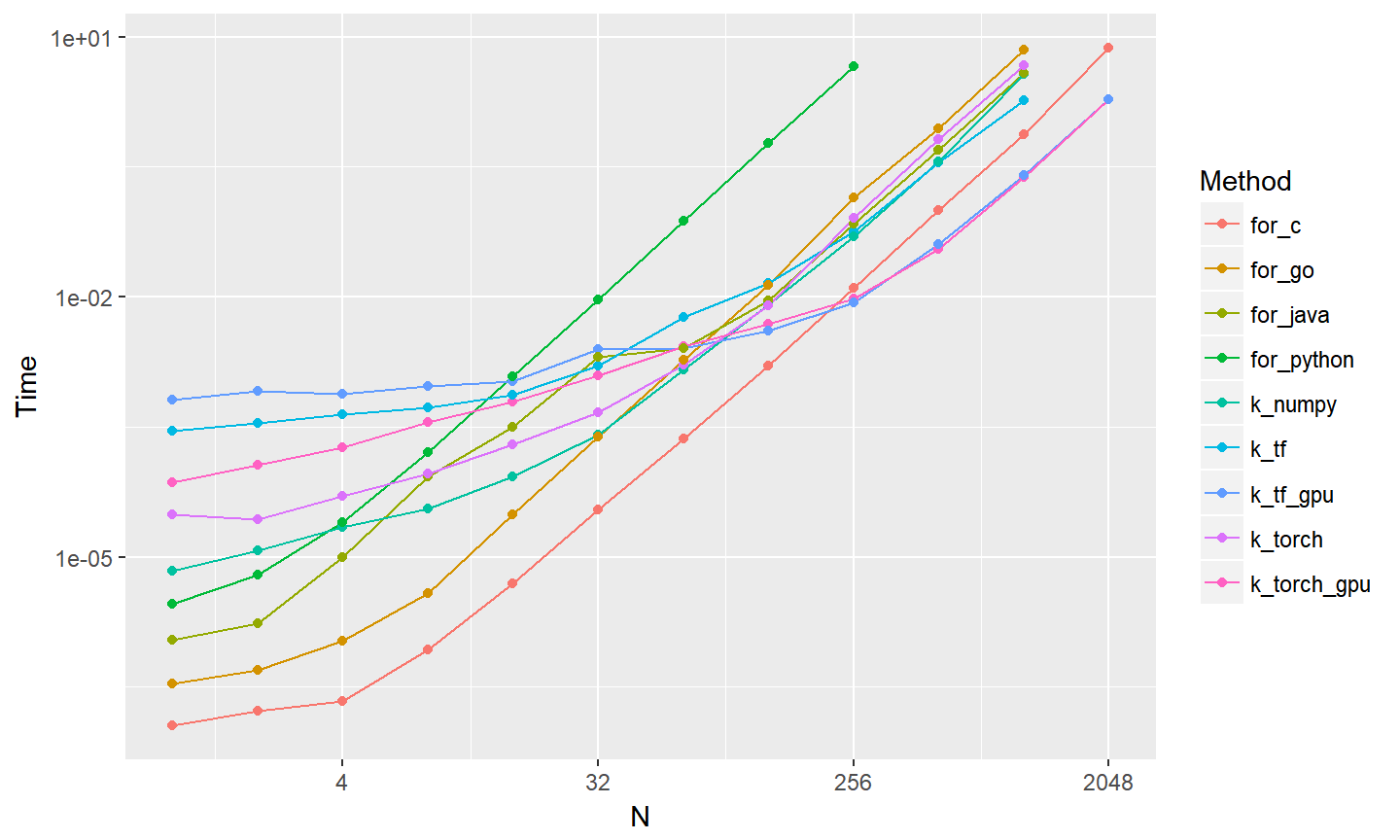## for

### for_python << for_go < for_java < for_c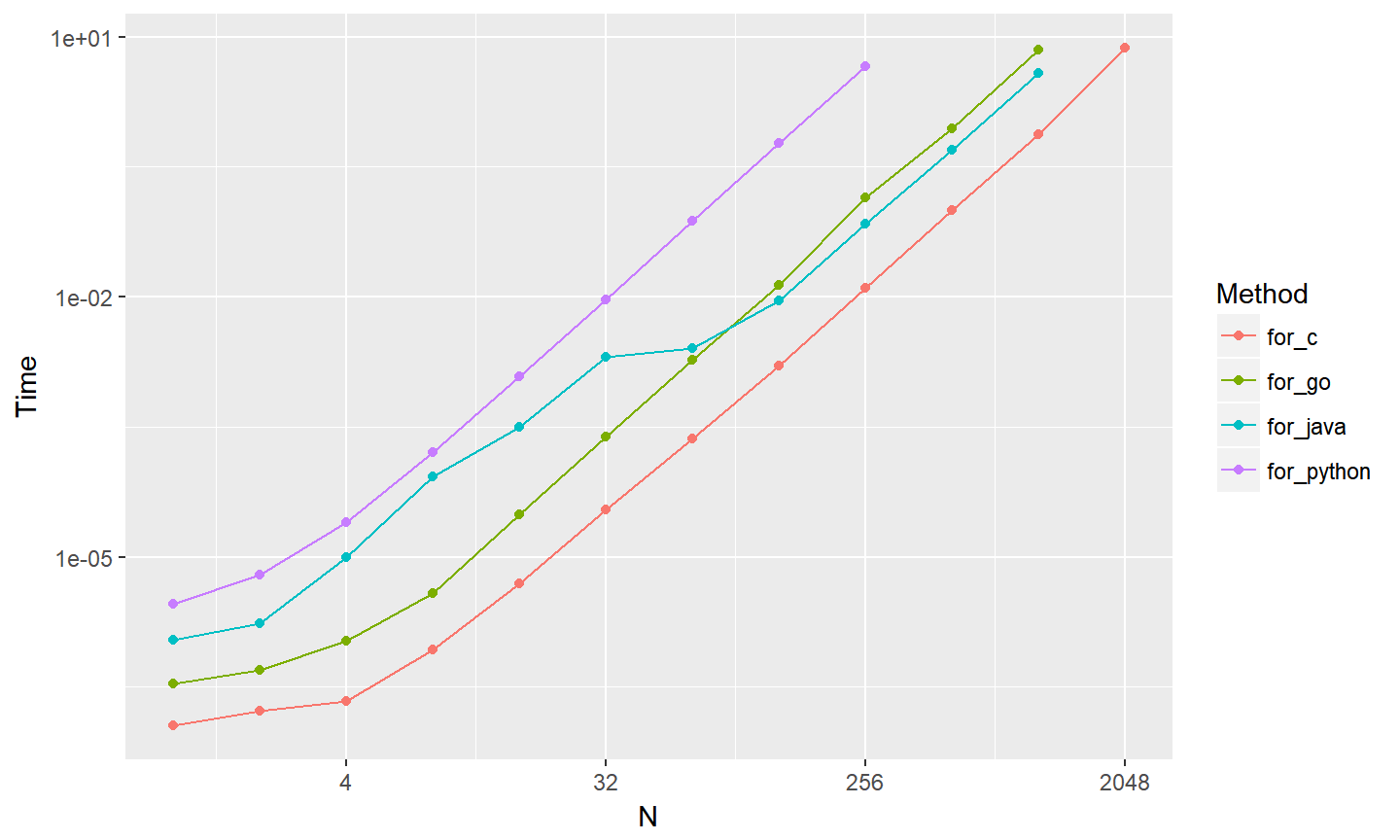## k_cpu

### k_numpy = k_tf = k_torch = for_java < for_c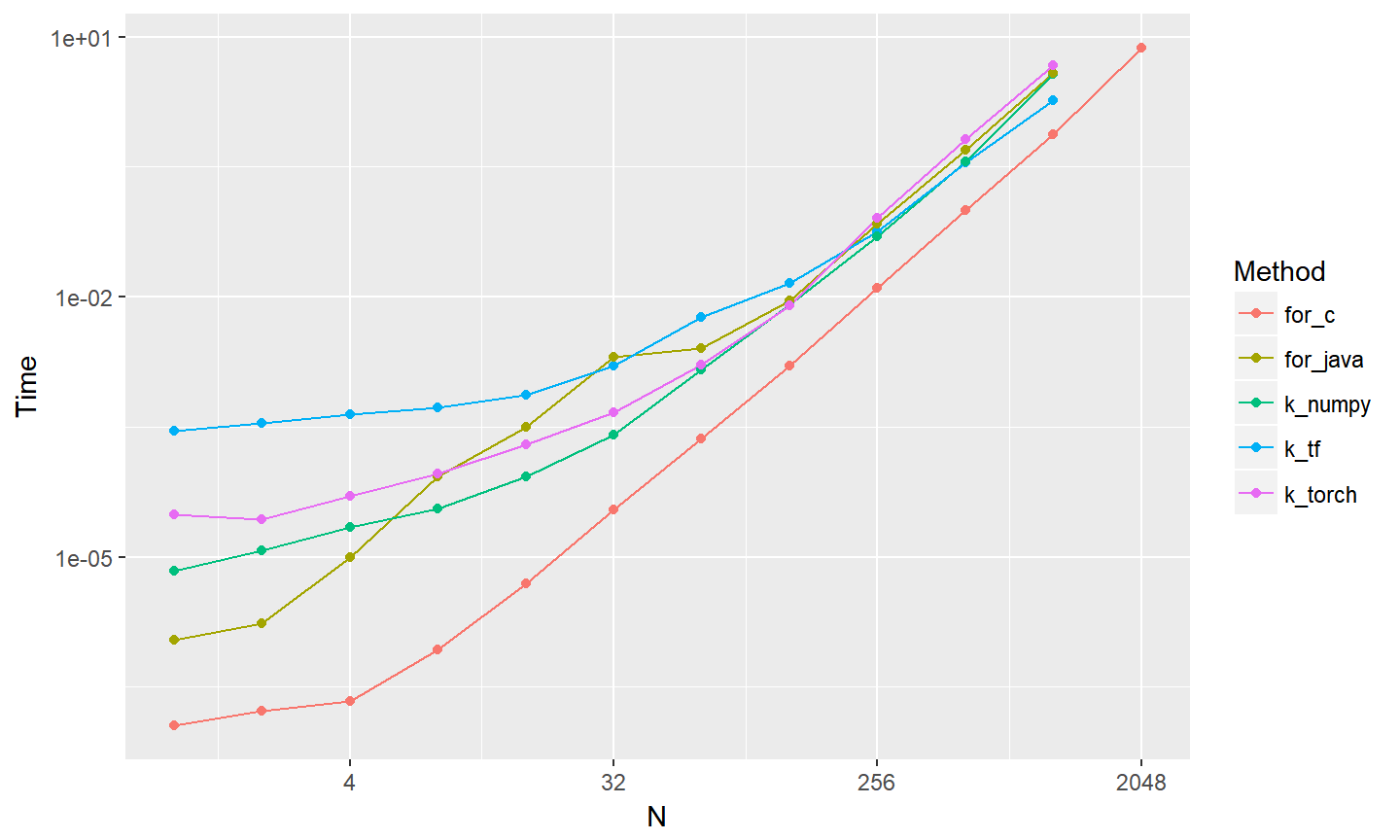## k_gpu

### for_java < for_c < k_tf_gpu = k_torch_gpu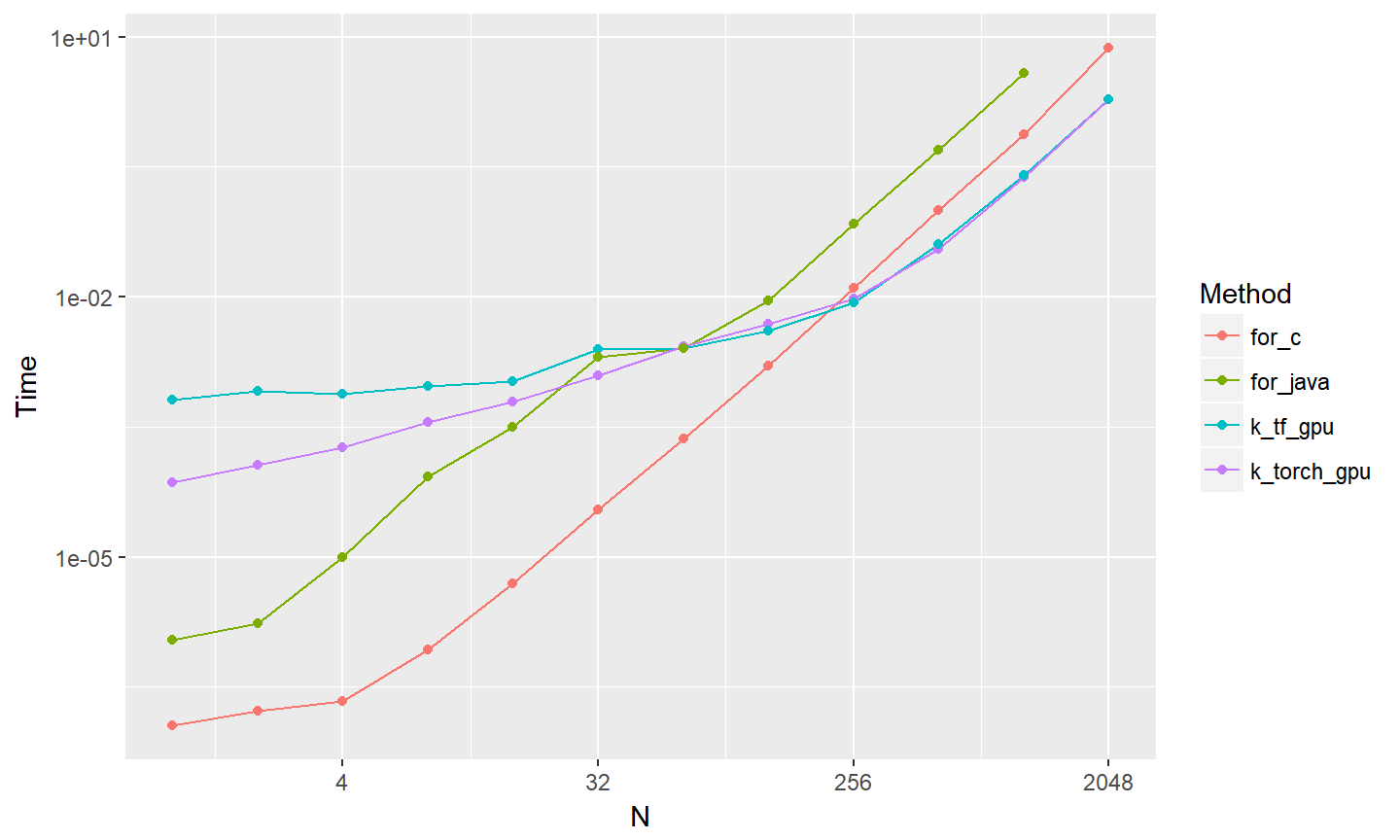## Matrix multiplication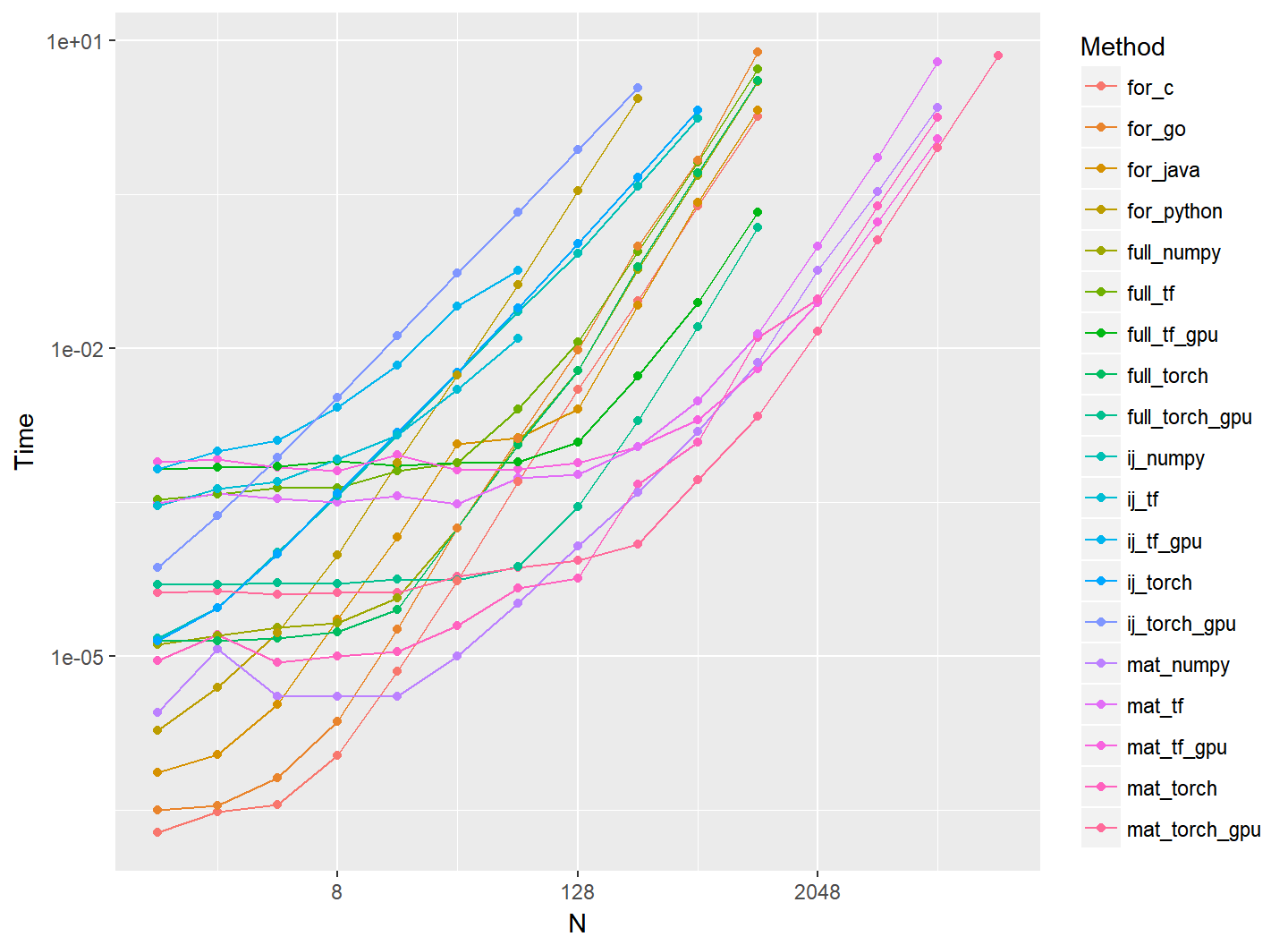## for

### for_python << for_go < for_java <= for_c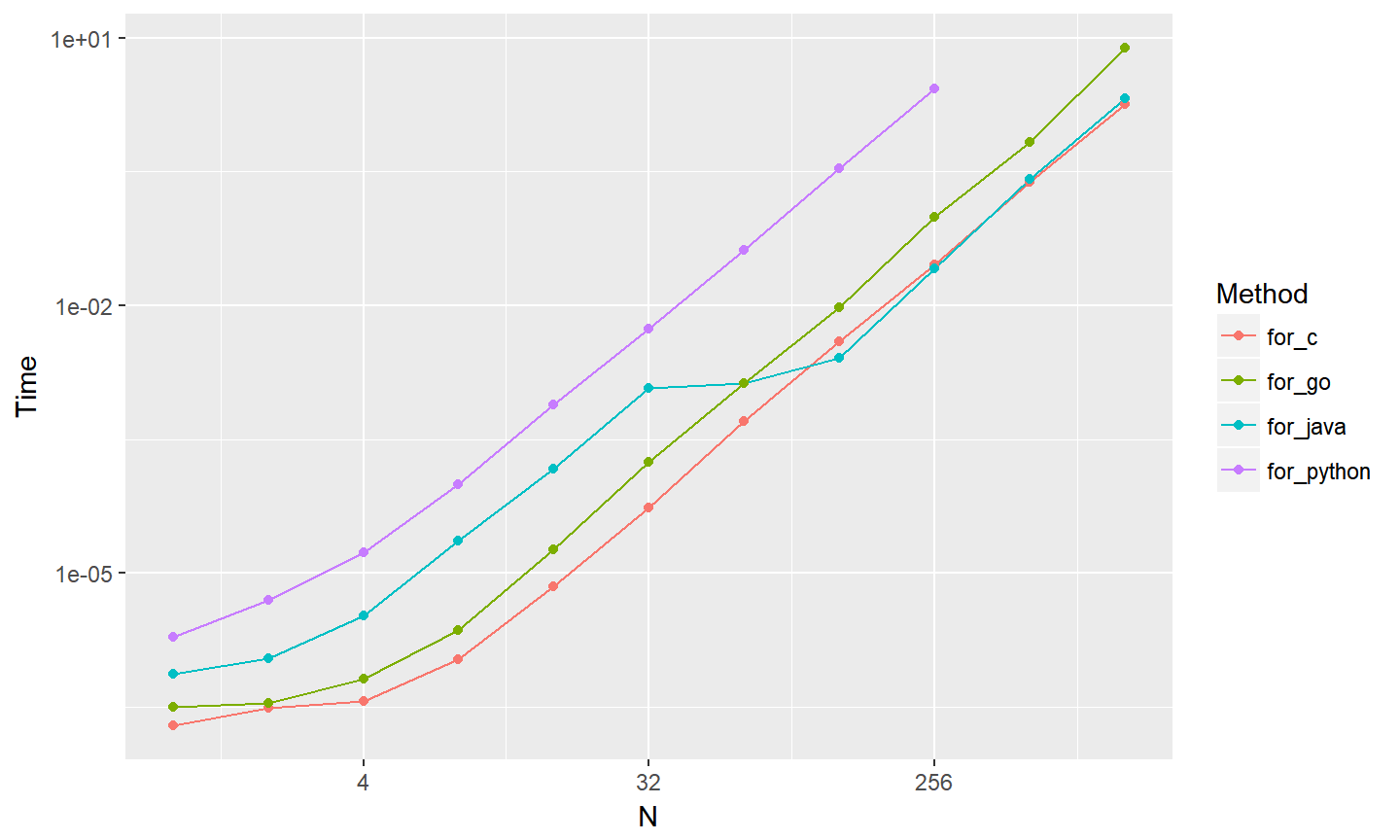## ij

### ij_*_gpu < ij_cpu < for_+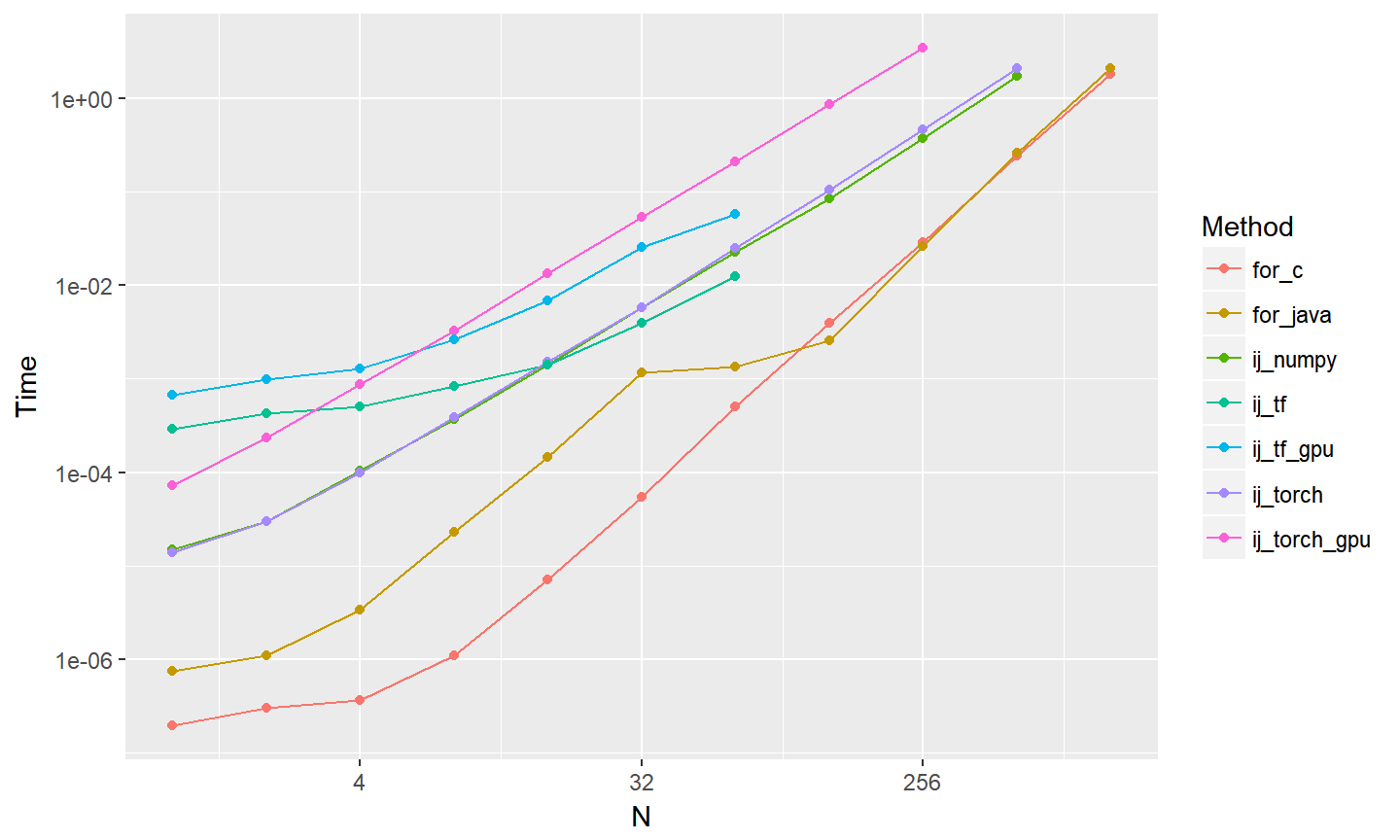## full

### full_cpu < for_+ < full_tf_gpu < full_torch_gpu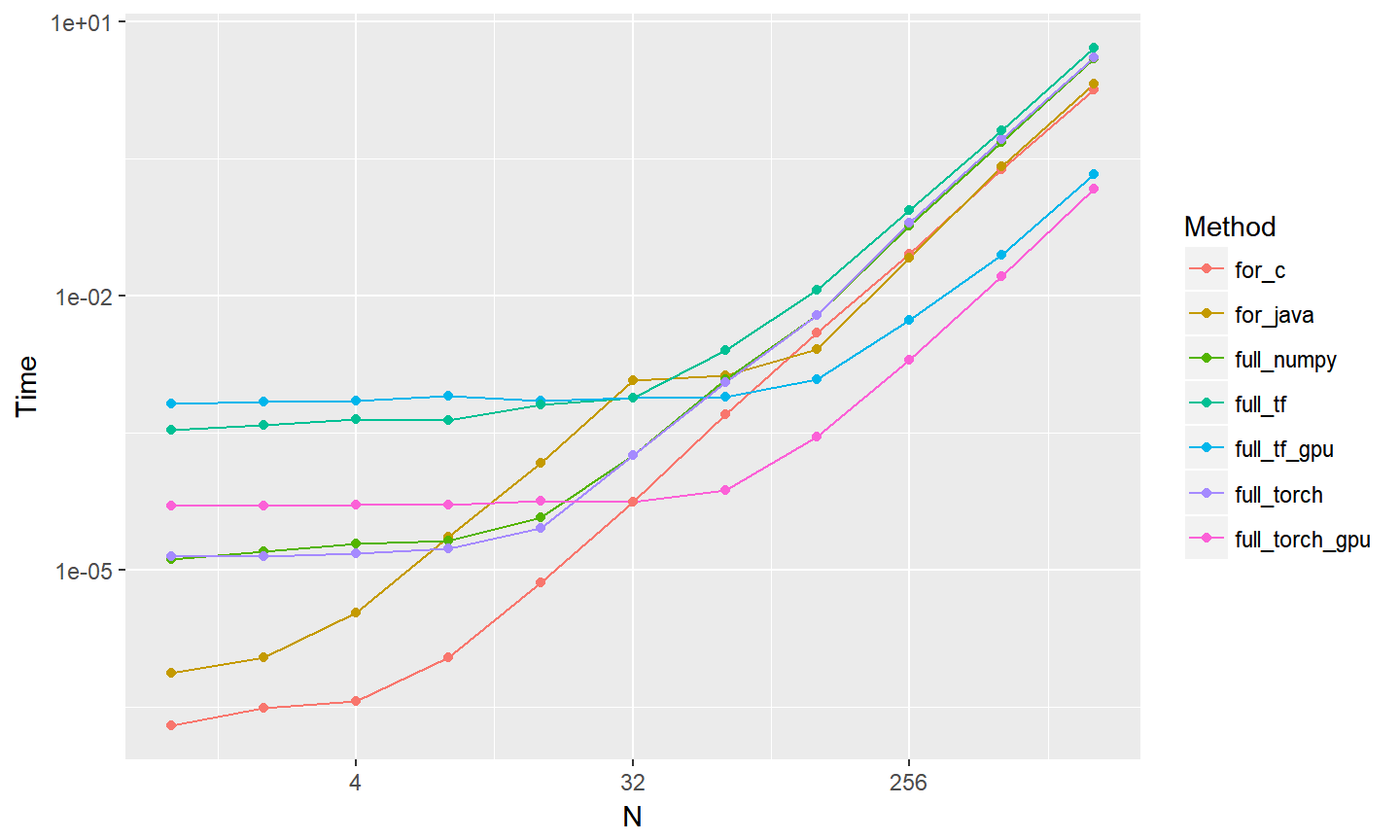## mat

### for_+ < full_*_gpu < mat_*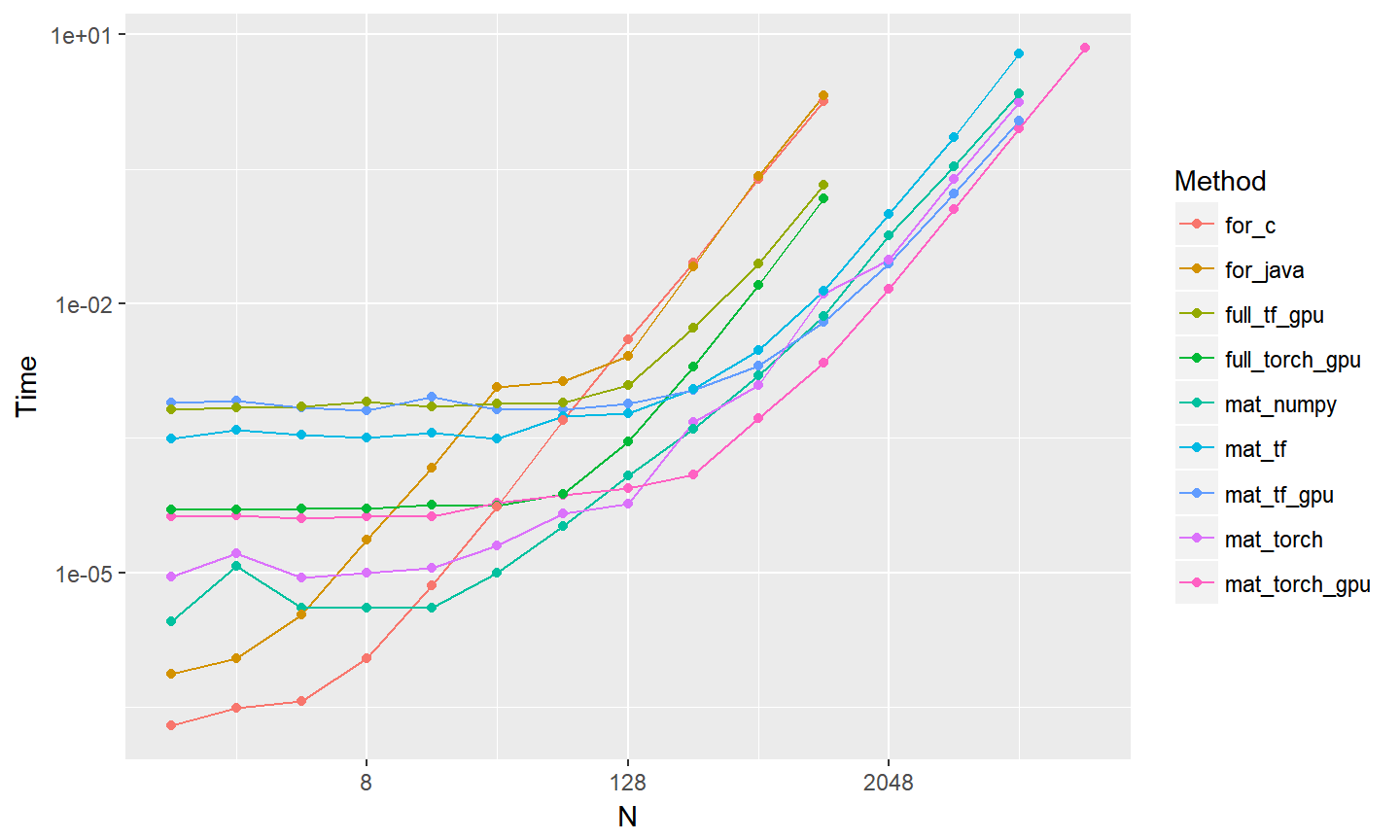## mat only

### tf < numpy < torch < tf_gpu < torch_gpu (mat_)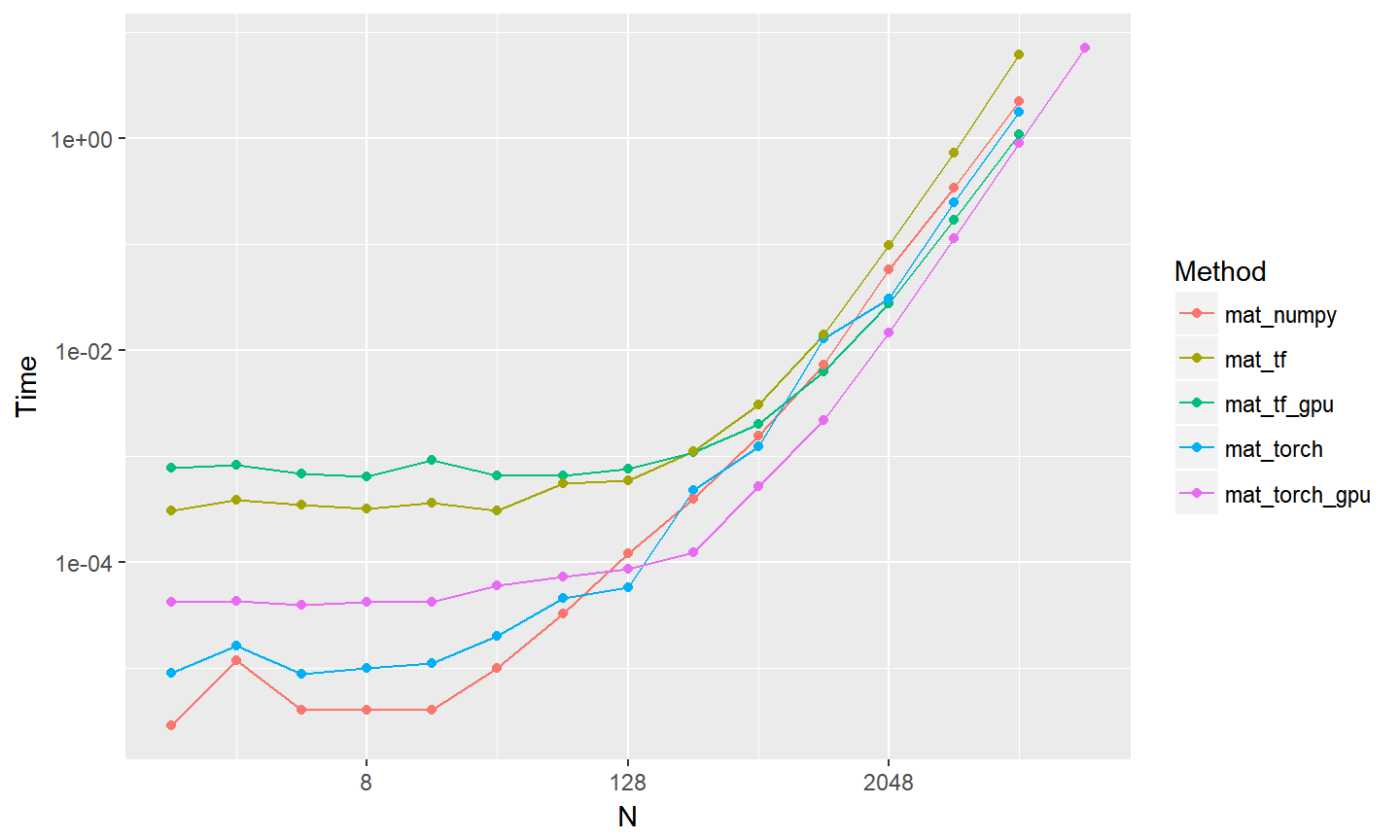## Summary

### Floyd-Warshall algorithm

• for | for_python << for_go < for_java < for_c
• k_cpu | k_numpy = k_tf = k_torch = for_java < for_c
• k_gpu | for_java < for_c < k_tf_gpu = k_torch_gpu
• I.e.
• for_python << for_go < k_numpy = k_tf = k_torch = for_java < for_c < k_tf_gpu = k_torch_gpu
• I.e.
• for_python << for_go < k_cpu = for_java < for_c < k_gpu

## Summary

### Matrix multiplication

• for | for_python << for_go < for_java <= for_c
• ij | ij_*_gpu < ij_cpu < for_+
• full | full_cpu < for_+ < full_tf_gpu < full_torch_gpu
• mat | for_+ < full_*_gpu < mat_*
• I.e.
• ij_gpu < ij_cpu < full_cpu < for_java <= for_c < full_tf_gpu < full_torch_gpu < mat_cpu < mat_gpu
• I.e.
• ij < full_cpu < for_java/c < full_gpu < mat_*